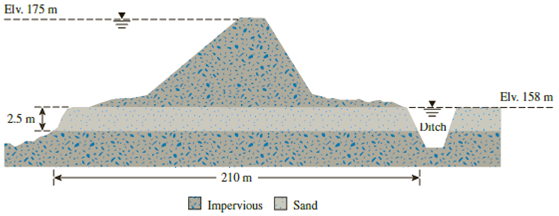Chapter 7, Problem 7.10PPrinciples of Geotechnical Enginee...

9th Edition
Braja M. Das + 1 other
ISBN: 9781305970939

Solutions

Chapter
SectionPrinciples of Geotechnical Enginee...

9th Edition
Braja M. Das + 1 other
ISBN: 9781305970939
Textbook Problem

Figure 7.32 shows the cross section of a levee which is 650 m long and is underlain by a 2.5-m-thick permeable sand layer. It was observed that the quantity of water flowing through the sand layer into the collection ditch is 13.5 m3/hr. What is the hydraulic conductivity of the sand layer?Figure 7.32

To determine

Find the hydraulic conductivity of the sand layer.

Explanation

Given information:

The length of levee l is 650 m.

The length of the seepage path L is 210 m.

The thickness of permeable sand layer t is 2.5 m.

The quantity of water flowing through the sand layer into the collection ditch q is 13.5m3/hr.

Calculation:

Determine the hydraulic gradient using the relation.

i=ΔhL

Substitute (175–158) m for Δh and 210 m for L.

i=(175158)210=0.081

Determine the hydraulic conductivity of the sand layer using the relation

Still sussing out bartleby?

Check out a sample textbook solution.

See a sample solution

The Solution to Your Study Problems

Bartleby provides explanations to thousands of textbook problems written by our experts, many with advanced degrees!

Get Started

What do we mean by dimensional homogeneity? Give an example.

Engineering Fundamentals: An Introduction to Engineering (MindTap Course List)

What is an assistive technology system?

Principles of Information Systems (MindTap Course List)

What is structural independence, and why is it important?

Database Systems: Design, Implementation, & Management

Convert 5 megawatts of power into BTU/hr, ft-lbs/s, and kJ/hr.

Fundamentals of Chemical Engineering Thermodynamics (MindTap Course List)

What e the main principles of source document design?

Systems Analysis and Design (Shelly Cashman Series) (MindTap Course List)

A scanner is a light-sensing output device.

Enhanced Discovering Computers 2017 (Shelly Cashman Series) (MindTap Course List)